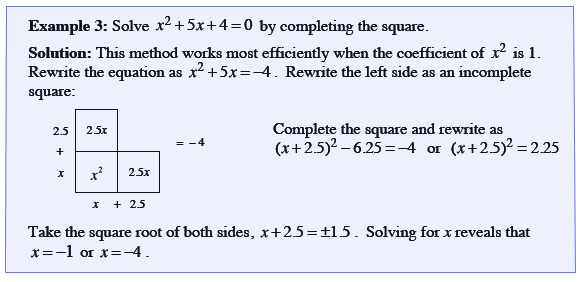Home > CCAA8 > Chapter cca9 > Lesson cca9.4.1 > Problem9-99

9-99.

Solve the equation below by completing the square. Give you answer in exact (radical) form. Homework Help ✎

$x^2−6x+3=0$

Use the Math Notes box.